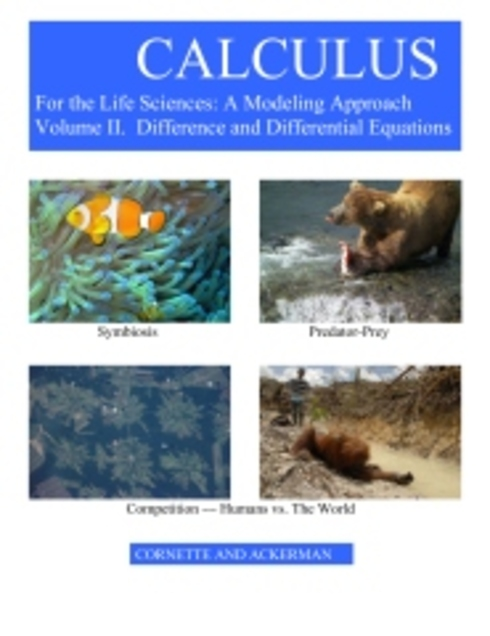# Calculus for the Life Sciences: A Modeling Approach Volume 2

(0 reviews)

No ratings

James L. Cornette, Iowa State University

Ralph A. Ackerman

Publisher: A.T. Still University

Language: English

## Conditions of UseAttribution-NonCommercial-NoDerivs
CC BY-NC-ND

• D Dynamical Equations from Volume I
• 13 Partial Derivatives
• 14 Difference Equation Models of Populations
• 15 Discrete Dynamical Systems
• 16 Nonlinear Dynamical Systems; Equilibria
• 17 Differential Equations
• 18 Second order, systems of two first order ODE’s

## Ancillary Material

• Submit ancillary resource

Our writing is based on three premises. First, life sciences students are motivated by and respond well to actual data related to real life sciences problems. Second, the ultimate goal of calculus in the life sciences primarily involves modeling living systems with difference and differential equations. Understanding the concepts of derivative and integral are crucial, but the ability to compute a large array of derivatives and integrals is of secondary importance. Third, the depth of calculus for life sciences students should be comparable to that of the traditional physics and engineering calculus course; else life sciences students will be short changed and their faculty will advise them to take the 'best' (engineering) course.

In our text, mathematical modeling and difference and differential equations lead, closely follow, and extend the elements of calculus. Chapter one introduces mathematical modeling in which students write descriptions of some observed processes and from these descriptions derive first order linear difference equations whose solutions can be compared with the observed data. In chapters in which the derivatives of algebraic, exponential, or trigonometric functions are defined, biologically motivated differential equations and their solutions are included. The chapter on partial derivatives includes a section on the diffusion partial differential equation. There are two chapters on non-linear difference equations and on systems of two difference equations and two chapters on differential equations and on systems of differential equation.